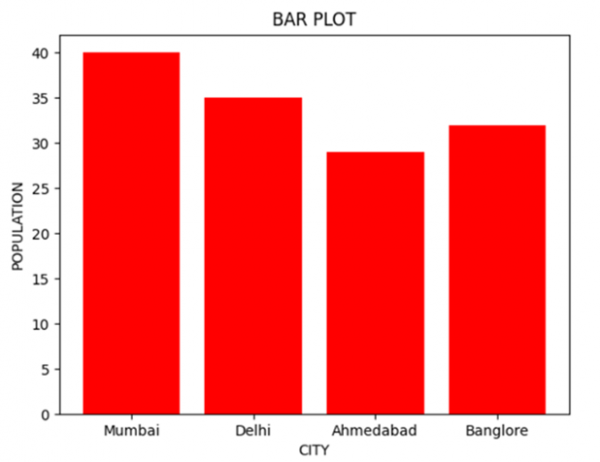# Displaying bar graphs using Matplotlib

In this program, we will plot a bar graph using the matplotlib library. The most important Step in solving matplotlib related problems using the matplotlib library is importing the matplotlib library. The syntax is −

import matplotlib.pyplot as plt

Pyplot is a collection of command style functions that make Matplotlib work like MATLAB

## Algorithm

Step 1: Define a list of values.
Step 2: Use the bar() function in the matplotlib.pyplot library and define different parameters like height, width, etc.
Step 3: Label the axes using xlabel() and ylabel().
Step 3: Plot the graph using show().

## Example Code

import matplotlib.pyplot as plt

data_x = ['Mumbai', 'Delhi', 'Ahmedabad', 'Banglore']
data_y = [40, 35, 29, 32]

plt.xlabel("CITY")
plt.ylabel("POPULATION")
plt.title("BAR PLOT")

plt.bar(data_x, data_y, color='red')
plt.show()

## OutputUpdated on: 16-Mar-2021

161 Views